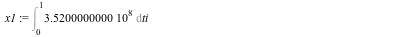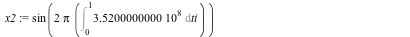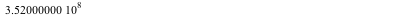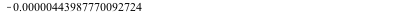:

## A bit of strangeness

Maple 2015

Recently I examined a piece of code of mine in an attempt to possibly convert it to another language as it is a numeric code and as such slower in Maple than I'd like it to run. In doing this I ran across the following strangeness, here reproduced in a minimum working example (file attached).

Consider this trivial integral:

x1:=Int(3.52*10^8, ti = 0 .. 1);(4)

and also this one:

x2:=sin(2*Pi*x1);(5)

I can then evaluate (4) and take sine(2Pi * the evaluation of (4)):

value(x1);(6)

sin(2*Pi*(6));(7)

Hmm... let's evaluate x2, which should be the same, right

value(x2);
0.00000556012229902952                                                              (8)

Oddly enough, it is not. Now the reason they are not 0 is due to round-off error (running the same sheet with Digits := 40 confirms that); but at the same time, (6) is in fact exact. More oddly, if I wrap the input leading to (7) in evalf() then it outputs 0., i.e. exact and correct. I suspect the problem must lie in the different treatments of Pi in the three cases.

I am not ready to call this behaviour a bug since I can see that different ways of evaluating what is essentially the same expression leads to a diffferent round-off. What strikes me is the relatively large errors in this case. The sheet was run with Digits being 15 (my default set in my .mapleinint), I initially expected somewhat more accuracy in the sine function than a mere 6 digits or so. On second thought, however, what is going on seems to be that the evaluation of the integral must be numerical and the large no. of cycles limits the accuracy; if one replaces 3.52E8 (a float) with 352E6 (an exact number) then (7) becomes 0 (exact) while (8) remains at the above value. Why

evalf(sin(2*Pi*(6)))

yields an exact value I do not quite understand.

So, caveat computor once again. This example, while it may look contrived, actually arose from a real-world case I was dealing with (the 352E6 is a frequency in Hz, in my actual application it can vary in time therefore the integration to get the no. of cycles in a given time interval). One annoyance here is that the "right" way to do this is not obvious, at least not to me.

M.D.

integration_test.mw﻿